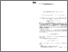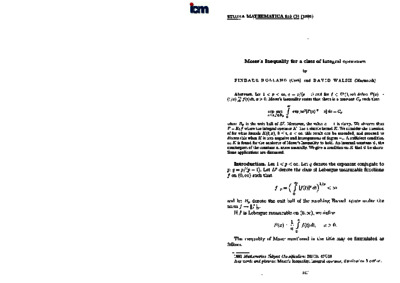# Moser's Inequality for a class of integral operators

Holland, Finbarr and Walsh, David (1995) Moser's Inequality for a class of integral operators. Studia Mathematica, 113 (2). pp. 141-166. ISSN 0039-3223Previewmore...Add this article to your Mendeley library

## Abstract

Let 1 < p < ∞, q = p/(p-1) and for f ∈ L p ( 0 , ∞ ) define F ( x ) = ( 1 / x ) ʃ 0 x f ( t ) d t , x > 0. Moser’s Inequality states that there is a constant C p such that s u p a ≤ 1 s u p f ∈ B p ʃ 0 ∞ e x p [ a x q | F ( x ) | q - x ] d x = C p where B p is the unit ball of L p . Moreover, the value a = 1 is sharp. We observe that F = K 1 f where the integral operator K 1 has a simple kernel K. We consider the question of for what kernels K(t,x), 0 ≤ t, x < ∞, this result can be extended, and proceed to discuss this when K is non-negative and homogeneous of degree -1. A sufficient condition on K is found for the analogue of Moser’s Inequality to hold. An internal constant ψ, the counterpart of the constant a, arises naturally. We give a condition on K that ψ be sharp. Some applications are discussed.

Item Type: Article Moser; Inequality; class; integral operators; Faculty of Science and Engineering > Mathematics and Statistics 14690 https://doi.org/10.4064/sm-113-2-141-168 Dr. David Walsh 11 Aug 2021 13:54 Studia Mathematica Polska Akademia Nauk YesItem control page